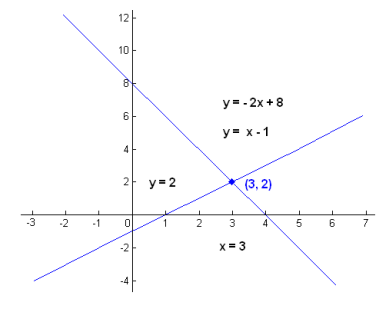# Systems of linear equations

Systems of linear equations (or linear systems as they are called sometimes) are defined as collections of linear equations that use the same set of variables. That means that within systems of linear equations you have two or more linear equations with the same variables. This is an example of such a system:

3x – 5y = 16

x – 3y = 8

This example shows a linear system with two equations and two variables. The number of equations in a system, as well as the number of variables, is not limited. But the number of solutions varies depending on the ratio of equations and variables in the system. If there are more variables then equations in a linear system, such a system has infinitely many solutions or sometimes unique sparse solutions. A system like that is called an underdetermined system. If a system has the same number of equations and variables, it has a single unique solution. But, if a system of linear equations has more equations than unknowns, it doesn’t have a solution. Such a system is called an overdetermined system.

Systems of linear equations are an important part of linear algebra and they play an important role in such sciences as engineering, physics, economics, chemistry and computer science, as well as modeling complex systems.

## Solving systems of linear equations

There are a few approaches to solving systems of linear equations. The simplest way is to use a method called substitution. This method is appropriate for the simplest kinds of linear systems and we will use this method to solve the system above.

The first step you need to perform when using substitution is to solve one of the equations for, let us say x in terms of y. We will do that with the second equation, since it only has one x.

x – 3y = 8

x = 8 + 3y

Now we insert the expression for x into the top equation:

3 * (8 + 3y) – 5y = 16

24 + 9y + -5y = 16

17y = 16 – 24

4y = -8 |: 4

y = -2

After this, we just insert the value of y into any of the given equations and we will have the value of x and the coordinates of the solution. So, let us insert the value of x into the second equation:

x – 3*(-2) = 8

x + 6 = 8

x = 8 – 6

x = 2

The coordinates of the solution are (2, -2). The solution is a single point in which two lines (which are the visual representation of the given equations in a coordinate system) cross each other.

## Graphing a system of linear equations

The solution to a system of linear equations can be determined graphically. The only thing you have to do is to draw the lines based on the given equations and then visually determine the point in which the lines cross paths. You can learn to do that by clicking on a link to our article on graphing linear equations.If you wish to practice solving and graphing systems of linear equations, please feel free to use the math worksheets below.

## Graphing systems of linear equations exams for teachers

 Exam Name File Size Downloads Upload date Graphing systems of linear equations Graphing systems of linear equations – Standard – very easy 833.1 kB 11906 September 3, 2019 Graphing systems of linear equations – Standard – easy 895.8 kB 8664 September 3, 2019 Graphing systems of linear equations – Standard – medium 809.8 kB 10850 September 3, 2019 Graphing systems of linear equations – Standard – hard 464.2 kB 6370 September 3, 2019 Graphing systems of linear equations – Standard – very hard 464.3 kB 4760 September 3, 2019 Graphing systems of linear equations – Slope/Intercept – very easy 833.1 kB 11906 September 3, 2019 Graphing systems of linear equations – Slope/Intercept – easy 895.8 kB 8664 September 3, 2019 Graphing systems of linear equations – Slope/Intercept – medium 846 kB 5911 September 3, 2019 Graphing systems of linear equations – Slope/Intercept – hard 464.5 kB 4454 September 3, 2019 Graphing systems of linear equations – Slope/Intercept – very hard 464.6 kB 3766 September 3, 2019 Systems of linear equations Systems of linear equations – very easy 461.3 kB 8432 September 3, 2019 Systems of linear equations – easy 461.8 kB 8371 September 3, 2019 Systems of linear equations – medium 462.2 kB 12539 September 3, 2019 Systems of linear equations – hard 462.4 kB 9486 September 3, 2019 Systems of linear equations – very hard 462.7 kB 8935 September 3, 2019

## Graphing systems of linear equations worksheets for students

 Worksheet Name File Size Downloads Upload date Graphing systems of linear equations – Standard 170.7 kB 9158 September 3, 2019 Graphing systems of linear equations – Slope/Intercept 221.8 kB 5389 September 3, 2019 Systems of linear equations 666.8 kB 12407 September 3, 2019